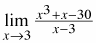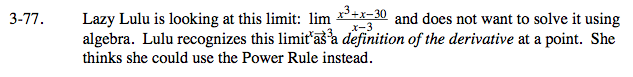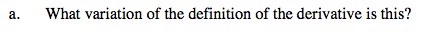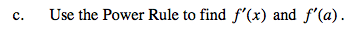### Home > CALC > Chapter Ch3 > Lesson 3.2.3 > Problem3-77

3-77.
1. Lazy Lulu is looking at this limit:and does not want to solve it using algebra. Lulu recognizes this limit as a definition of the derivative at a point. She thinks she could use the Power Rule instead. Homework Help ✎

1. What variation of the definition of the derivative is this?

2. What is f(x) ? What is a?

3. Use the Power Rule to find f ′(x) and f ′(a).This is Ana's Definition of the Derivative:

$f'(a)=\lim_{x\rightarrow a}\frac{f(x)-f(a)}{x-a}$

$=\lim_{x\rightarrow 3}\frac{(x^{3}+x)-((3)^{3}+3)}{x-3}=\lim_{x\rightarrow 3}\frac{x^{3}+x-30}{x-3}$Observe that x3 + x = 30 when x = 3.

f(x) = x3 + 3

a = 3
Notice that f(3) = 3³ + 3 = 30, which confirms that equation of f(x) is correct.f(x) = x³ + x
f '(x) =_______________________
f '(3) =__________
You just avoided a lot of Algebra!